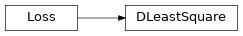# DLeastSquare¶

Inheritance Diagramclass ashpy.keras.losses.DLeastSquare[source]

Bases: tensorflow.python.keras.losses.Loss

Discriminator Least Square Loss as tf.keras.losses.Loss.

Methods

 __init__() Least square Loss for Discriminator. call(d_real, d_fake) Compute the Least Square Loss.

Attributes

 reduction Return the reduction type for this loss.
__init__()[source]

Least square Loss for Discriminator.

Reference: Least Squares Generative Adversarial Networks  .

Basically the Mean Squared Error between the discriminator output when evaluated in fake samples and 0 and the discriminator output when evaluated in real samples and 1: For the unconditioned case this is:

$L_{D} = \frac{1}{2} E[(D(x) - 1)^2 + (0 - D(G(z))^2]$

where x are real samples and z is the latent vector.

For the conditioned case this is:

$L_{D} = \frac{1}{2} E[(D(x, c) - 1)^2 + (0 - D(G(c), c)^2]$

where c is the condition and x are real samples.

  Least Squares Generative Adversarial Networks https://arxiv.org/abs/1611.04076
Return type: None
call(d_real, d_fake)[source]

Compute the Least Square Loss.

Parameters: d_real (tf.Tensor) – Discriminator evaluated in real samples. d_fake (tf.Tensor) – Discriminator evaluated in fake samples. Tensor tf.Tensor – Loss.
reduction

Return the reduction type for this loss.

Return type: ReductionV2 tf.keras.losses.Reduction – Reduction.